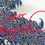# One-Dimensional Kinematics: Motion Along a Straight Line

The First Step: Choosing Coordinates

Before beginning a problem in kinematics, you must set up your coordinate system. In one-dimensional kinematics, this is simply an x-axis and the direction of the motion is usually the positive-x direction. Though displacement, velocity, and acceleration are all vector quantities, in the one-dimensional case they can all be treated as scalar quantities with positive or negative values to indicate their direction.

The positive and negative values of these quantities are determined by the choice of how you align the coordinate system.

Velocity:

Velocity represents the rate of change of displacement over a given amount of time. The displacement in one-dimension is generally represented in regards to a starting point of x1 and x2. The time that the object in question is at each point is denoted as t1 and t2 (always assuming that t2 is later than t1, since time only proceeds one way). The change in a quantity from one point to another is generally indicated with the Greek letter delta, Δ.

Using these notations, it is possible to determine the average velocity (vav) in the following manner:

vav = (x2-x1) / (t2-t1)=Δx/Δt If you apply a limit as Δt approaches 0, you obtain an instantaneous velocity at a specific point in the path. Such a limit in calculus is the derivative of x with respect to t, or dx/dt. Acceleration

Acceleration represents the rate of change in velocity over time. Using the terminology introduced earlier, we see that the average acceleration (aav) is: aav = (v2 - v1) / (t2 - t1) = Δx / Δt

Again, we can apply a limit as Δt approaches 0 to obtain an instantaneous acceleration at a specific point in the path. The calculus representation is the derivative of v with respect to t, or dv/dt. Similarly, since v is the derivative of x, the instantaneous acceleration is the second derivative of x with respect to t, or d2x/dt2. Constant Acceleration

In several cases, such as the Earth's gravitational field, the acceleration may be constant - in other words the velocity changes at the same rate throughout the motion. Using our earlier work, set the time at 0 and the end time as t (picture starting a stopwatch at 0 and ending it at the time of interest). The velocity at time 0 is v0 and at time t is v, yielding the following two equations:

a = (v - v0)/(t - 0) v = v0 + at

Applying the earlier equations for vav for x0 at time 0 and x at time t, and applying some manipulations (which I will not prove here), we get: x = x0 + v0t + 0.5at2 v2 = v02 + 2a(x - x0)

x - x0 = (v0 + v)t / 2

The above equations of motion with constant acceleration can be used to solve any kinematic problem involving motion of a particle on a straight line with constant acceleration.5 years, 11 months ago

This discussion board is a place to discuss our Daily Challenges and the math and science related to those challenges. Explanations are more than just a solution — they should explain the steps and thinking strategies that you used to obtain the solution. Comments should further the discussion of math and science.

When posting on Brilliant:

• Use the emojis to react to an explanation, whether you're congratulating a job well done , or just really confused .
• Ask specific questions about the challenge or the steps in somebody's explanation. Well-posed questions can add a lot to the discussion, but posting "I don't understand!" doesn't help anyone.
• Try to contribute something new to the discussion, whether it is an extension, generalization or other idea related to the challenge.

MarkdownAppears as
*italics* or _italics_ italics
**bold** or __bold__ bold
- bulleted- list
• bulleted
• list
1. numbered2. list
1. numbered
2. list
Note: you must add a full line of space before and after lists for them to show up correctly
paragraph 1paragraph 2

paragraph 1

paragraph 2

[example link](https://brilliant.org)example link
> This is a quote
This is a quote
    # I indented these lines
# 4 spaces, and now they show
# up as a code block.

print "hello world"
# I indented these lines
# 4 spaces, and now they show
# up as a code block.

print "hello world"
MathAppears as
Remember to wrap math in $$ ... $$ or $ ... $ to ensure proper formatting.
2 \times 3 $2 \times 3$
2^{34} $2^{34}$
a_{i-1} $a_{i-1}$
\frac{2}{3} $\frac{2}{3}$
\sqrt{2} $\sqrt{2}$
\sum_{i=1}^3 $\sum_{i=1}^3$
\sin \theta $\sin \theta$
\boxed{123} $\boxed{123}$